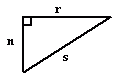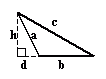Email us to get an instant 20% discount on highly effective K-12 Math & English kwizNET Programs!

#### Online Quiz (WorksheetABCD)

Questions Per Quiz = 2 4 6 8 10

### MEAP Preparation - Grade 5 Mathematics3.3 Classifying Triangles by Angles

#### Classification of triangles based on angles

1. Acute angled triangle:

• If all the angles of a triangle are acute angles, then it is called an acute angled triangle.

•2. Right angled triangle:
• A triangle in which one of the angles is a right angle is called a right angled triangle.

• In a right angled triangle, the side opposite to the right angle is called its hypotenuse.

•3. Obtuse angled triangle:
• A triangle containing an obtuse angle is called an obtuse angled triangle.

•Directions: Read the above properties of triangles and answer the following questions:
1. Illustrate each of the above properties by drawing a triangle.
2. Write in your own words, how to classify triangles according to their angles.

 Q 1: The angles 90 degrees, 30 degrees and 60 degrees represents an obtuse angled triangle.FalseTrue Q 2: The angles 70 degrees, 80 degrees and 30 degrees represents the angles of an acute angled triangle.TrueFalse Q 3: The side opposite to the right angle in a right angled triangle is called the ________ of the triangle.Answer: Q 4: A triangle whose angles are given by 75 degrees, 45 degrees and 60 degrees is a (an) ________ angled triangle.Answer: Q 5: In a triangle the angles are given by 55 degrees, 25 degrees and 100 degrees. The triangle is a (an) ________.Acute angled scalene triangleRight angled scalene triangleObtuse angled scalene triangle Q 6: A (an) ________ angled triangle has its angle measures as 100 degrees, 60 degrees and 20 degrees.Answer: Q 7: The angles 30 degrees, 60 degrees and 90 degrees represents a (an) ________ angled triangle.Answer: Q 8: The angles 90 degrees, 45 degrees and 45 degrees represents a (an) ________ triangle.Right angled isoscelesAcute angled isoscelesObtuse angled scalene Question 9: This question is available to subscribers only! Question 10: This question is available to subscribers only!